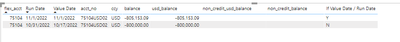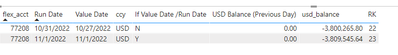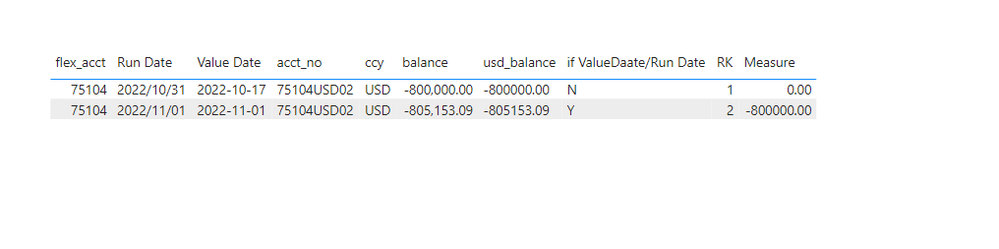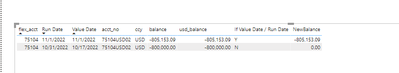cancel
Showing results for
Did you mean:Helper III

## Formula to pull in previous day balanceHello,

I am looking to pull the previous run date (second column) USD balance (7th column) if the "if value date/Run date" column is equal to "Y".

For example, the new column should contain a value of -800,000 (previous day USD balance) in the first row and in the second row a value of 0 since the if statement is equal to "N".  Please note, this should be applied by flex_acct codes as there are many different codes (ex:75104, 75015) on the report (first column)

Also, this report runs 5 days a week and therefore does not include weekend dates so it would need to find the previous run date and not previous date.

1 ACCEPTED SOLUTIONCommunity Support

Hi @gmasta1129 ,

``````Measure =
VAR cur_rk = [RK]
VAR cur_flexacct =
SELECTEDVALUE ( 'Table'[flex_acct] )
VAR cur_cd =
SELECTEDVALUE ( 'Table'[if ValueDaate/Run Date] )
VAR tmp =
FILTER (
ALL ( 'Table' ),
'Table'[flex_acct] = cur_flexacct
&& [RK] = cur_rk - 1
)
VAR _val =
CALCULATE ( MAX ( 'Table'[usd_balance] ), tmp )
VAR cur_rd =
SELECTEDVALUE ( 'Table'[Run Date] )
VAR _pre_date =
CALCULATE ( MAX ( 'Table'[Run Date] ), tmp )
VAR cd =
DATEDIFF ( cur_rd, _pre_date, DAY )
RETURN
IF ( DAY ( cur_rd ) = 1 && cd <> 1, 0, IF ( cur_cd = "N", 0, _val ) )
``````Please refer the attached .pbix file.

Best regards,
Community Support Team_ Binbin Yu
If this post helps, then please consider Accept it as the solution to help the other members find it more quickly.

8 REPLIES 8Helper III

@v-binbinyu-msft The formula above is pulling all zeros even when there is a balance in the previous day.

I think the issue is the return formula.

RETURN IF ( DAY ( cur_rd ) = 1 && cd <> 1, 0, IF ( cur_cd = "N", 0, _val ) )

` `

It is correct in this scenario since the last negative balance was on 8/2 and there was no balance on 10/31But this scenario has a balance as of 10/31 (previous day) and it is pulling 0 when I should be seeing -3.8 millionHelper III

Hello @v-binbinyu-msft ,

This worked exactly as expected except for one scenario.  If there is a zero balance for a certain run date in a specific flex acct then the flex acct does not pull onto the report.

Therefore the formula is finding the latest balance from the latest run date and pulling that value in.  Can the formula be tweaked where it finds the previous "Run Date" balance and if there is no balance,  then pull in 0?

For example, in the screenshot below, flex_acct 71513, the USD balance on run date 11/1/2022 is  -8,785.07 but there is no run date for 10/31/2022. The last run date is 10/24/2022 which means the balance as of 10/31/2022 is 0. For our reporting purposes any flex acct with a zero balance does not pull into the report.

Your formula which is under column "USD Balance (Previous Day)" is pulling in the latest run date balance which is 10/24/2022 of -999,519,51. This is incorrect since the previous day from 11/1/2022 is 10/31/2022.  because the balance is 0 on 10/31, you will not see a line on the report for it.  it does not pull in. I would be expecting to see 0 instead of -991,529.51.Community Support

Hi @gmasta1129 ,

``````Measure =
VAR cur_rk = [RK]
VAR cur_flexacct =
SELECTEDVALUE ( 'Table'[flex_acct] )
VAR cur_cd =
SELECTEDVALUE ( 'Table'[if ValueDaate/Run Date] )
VAR tmp =
FILTER (
ALL ( 'Table' ),
'Table'[flex_acct] = cur_flexacct
&& [RK] = cur_rk - 1
)
VAR _val =
CALCULATE ( MAX ( 'Table'[usd_balance] ), tmp )
VAR cur_rd =
SELECTEDVALUE ( 'Table'[Run Date] )
VAR _pre_date =
CALCULATE ( MAX ( 'Table'[Run Date] ), tmp )
VAR cd =
DATEDIFF ( cur_rd, _pre_date, DAY )
RETURN
IF ( DAY ( cur_rd ) = 1 && cd <> 1, 0, IF ( cur_cd = "N", 0, _val ) )
``````Please refer the attached .pbix file.

Best regards,
Community Support Team_ Binbin Yu
If this post helps, then please consider Accept it as the solution to help the other members find it more quickly.Community Support

Hi @gmasta1129 ,

1. below is my test table

Table:2. create measure with below dax formula

``````RK =
VAR cur_flexacct =
SELECTEDVALUE ( 'Table'[flex_acct] )
VAR tmp =
FILTER ( ALL ( 'Table' ), 'Table'[flex_acct] = cur_flexacct )
RETURN
RANKX ( tmp, CALCULATE ( MAX ( 'Table'[Run Date] ) ),, ASC, DENSE )
``````
``````Measure =
VAR cur_rk = [RK]
VAR cur_flexacct =
SELECTEDVALUE ( 'Table'[flex_acct] )
VAR cur_cd =
SELECTEDVALUE ( 'Table'[if ValueDaate/Run Date] )
VAR tmp =
FILTER (
ALL ( 'Table' ),
'Table'[flex_acct] = cur_flexacct
&& [RK] = cur_rk - 1
)
VAR _val =
CALCULATE ( MAX ( 'Table'[usd_balance] ), tmp )
RETURN
IF ( cur_cd = "N", 0, _val )
``````

3. add a table visual with fields and measurePlease refer the attached .pbix file.

Best regards,
Community Support Team_ Binbin Yu
If this post helps, then please consider Accept it as the solution to help the other members find it more quickly.Helper III

I entered your formula and the first row is pulling in -805,000 instead of -800,000.

Please see screenshot below for reference.Resident Rockstar

Hi @gmasta1129 ,

I don't think I understand your requirements. Here is what I was trying to show:

For example, the new column should contain a value of -800,000 (previous day USD balance) in the first row and in the second row a value of 0 since the if statement is equal to "N".Helper III

Hello @djurecicK2,

Thank you for the quick response but I would need the previous day balance to pull into the column.  Threfore 10/31/2022 run date balance of 800,000 should pull into the first row and 0 should pull into the second row.Resident Rockstar

Hi @gmasta1129 ,

Here is a way to do that in DAX by creating a new column:

NewBalance = IF('Table'[RunDate]='Table'[ValueDate],'Table'[Balance],0)Announcements#### Exclusive opportunity for Women!

Join us for a free, hands-on Microsoft workshop led by women trainers for women where you will learn how to build a Dashboard in a Day!#### Power Platform Conference-Power BI and Fabric Sessions

Join us Oct 1 - 6 in Las Vegas for the Microsoft Power Platform Conference.Top Solution Authors
Top Kudoed Authors
Users online (2,887)